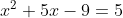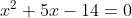Scan QR code or get instant email to install app

Question:

# 1212Which of the following values of x satisfies the equation above?

A -7
explanation

1212Subtracting 5 from both sides of the equation gives. The left-hand side of the equation can be factored, giving (x + 7)(x − 2) = 0. Therefore, the solutions to the quadratic equation are x = −7 and x = 2.